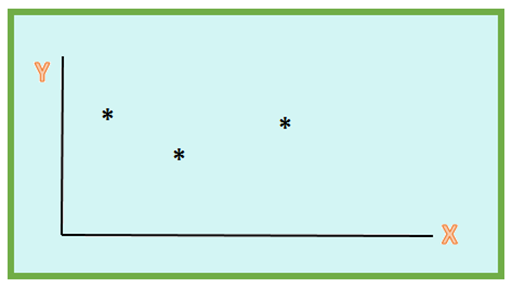Home » Subject » Management » Notes » Simple correlation and regression

# Simple Correlation and Regression

Regression and correlation analysis are statistical techniques that are broadly used in physical geography to examine causal relationships between variables. Regression and correlation measure the degree of relationship between two or more variables in two different but related ways.

## Correlation

Correlation is a statistical method used to determine the extent to which two variables are related. Correlation analysis measures the degree of association between two or more variables. Parametric methods of correlation analysis assume that for any pair or set of values taken under a given set of conditions, variation in each of the variables is random and follows a normal distribution pattern. In scattered diagram, following elements are represented:
1. Rectangular coordinate
2. Two quantitative variables
3. One variable is called independent (X) and the second is called dependent (Y)
4. Points are not joined
5. No frequency tableIn correlation analysis, statisticians estimate a sample correlation coefficient, more specifically the Pearson Product Moment correlation coefficient. Correlation coefficient is the Statistic showing the degree of relation between two variables. The simple correlation coefficient, denoted r, ranges between -1 and +1 and quantifies the direction and strength of the linear association between the two variables. It is also called Pearson's correlation or product moment correlation coefficient. It measures the nature and strength between two variables of the quantitative type.

In scatter plot, the pattern of data is indicative of the type of relationship between two variables. It may be

1. Positive relationship
2. Negative relationship
3. No relationship

The sign of the correlation coefficient indicates the direction of the association. The magnitude of the correlation coefficient indicates the strength of the association.

## Regression

Regression analysis encompass to identify the relationship between a dependent variable and one or more independent variables. Regression calculates the "best-fit" line for a certain set of data. The regression line makes the sum of the squares of the residuals smaller than for any other line. In regression analysis, a single dependent variable, Y, is considered to be a function of one or more independent variables, X1, X2, and so on. The values of both the dependent and independent variables are assumed as being determined in an error-free random manner.

Additionally, parametric forms of regression analysis undertake that for any given value of the independent variable, values of the dependent variable are normally distributed about some mean. Application of this statistical procedure to dependent and independent variables produces an equation that "best" approximates the functional relationship between the data observations.

A model of the relationship is hypothesized, and estimates of the parameter values are used to develop an estimated regression equation. Various tests are then employed to determine if the model is satisfactory. If the model is thought satisfactory, the estimated regression equation can be used to predict the value of the dependent variable given values for the independent variables.

In a simple regression analysis, one dependent variable is measured in relation to only one independent variable. The analysis is designed to develop an equation for the line that best models the relationship between the dependent and independent variables. This equation has the mathematical form:

Y = a + bX

In above equation, Y is the value of the dependent variable, X is the value of the independent variable is the intercept of the regression line on the Y axis when X = 0, and b is the slope of the regression line.

 Correlation Linear Regression Correlation examines the relationship between two variables using a standard unit. However most applications use row units as on input. Regression examines the relationship between one dependent variable and one or more independent variables. Calculations may use either row unit values, or standard units as input. The calculation is symmetrical, meaning that the order of comparison does not change the result. The calculation is not symmetrical. So one variable is assigned the dependent role(the value being predicted) and one are more the dependent role(the values hypothesize to impact the dependent variables). Correlation coefficients indicate the strength of a relationship. Regression shows the effect of one unit change in an independent variable on the dependent variable. Correlation removes the effect of different measurement scales. Therefore, comparison between different models is possible since the rho coefficient is in standardized units. Linear regression using row unit measurement scales can be used to predict outcomes. For example, if a model shows that spending more money on advertising will increase sales, then one can say that for every added \$ in advertising, sales wil increase by β.

To summarize, Correlation is a specific procedures from a larger family of regression procedures. Regression procedures scrutinise the relationship between two or more sets of paired variables. Correlation and regression analysis are linked in a way that both deal with relationships among variables.